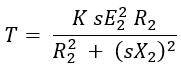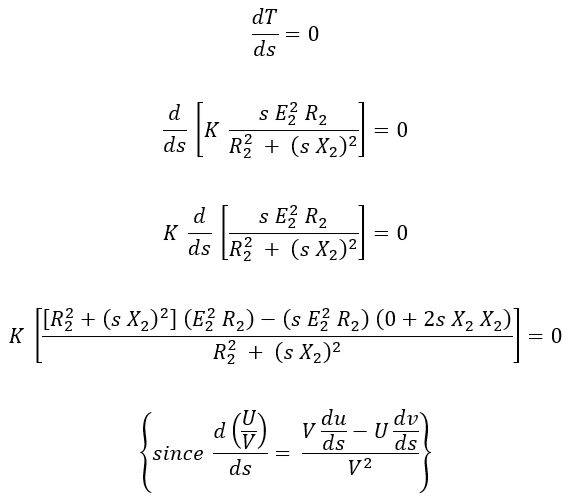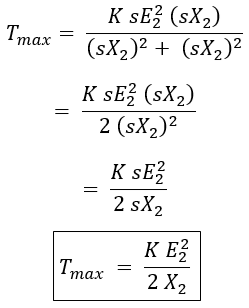# Maximum Torque Condition of Induction Motor & Expression

In the last article, we have seen the torque equation of induction motor i.e., the amount of torque produced on the rotor depends upon rotor current, rotor resistance, rotor reactance, and slip.

From the torque-slip characteristics of the induction motor, it can be noticed that the torque of the motor is directly proportional to the slip until the motor attains its maximum torque Tmax i.e., at full load.

Once, the amount of load on the motor meets that it has produced its maximum torque, thereby we can see an inverse relation (inverse proportionality) between torque and slip for any further increase in load. The below figure shows the effect of change of load on an induction motor.

Let's see the condition and expression for obtaining the maximum torque in an induction motor.

## Maximum Torque Condition of an Induction Motor :

#### The expression for torque produced in an induction motor under the running condition is given as,In an induction motor, to obtain maximum torque condition, differentiate the equation of torque with respect to slip (as slip is the variable quantity with respect to torque) and equate it to zero.Therefore,Where,R2 = Rotor resistanceX2 = Rotor reactances = Slip of the motor.

Therefore, the torque produced is maximum when the resistance of the rotor is equal to slip times rotor reactance or we can say under the running condition the torque produced will be maximum when rotor resistance is equal to rotor reactance. From the above condition, the slip 'sm' at which the torque is maximum is given by,

## Expression for Maximum Torque :

#### The expression for maximum torque can be obtained by substituting R2 = sX2 in the torque equation. The equation of torque is,Substituting R2 = sX2 in the above equation,From the expression of Tmax, it can be observed that,There is no relation between maximum torque and rotor resistance (independent of rotor resistance).But here the rotor reactance is determined by the rotor resistance. Hence maximum torque also depends upon rotor resistance.Maximum torque is inversely proportional to rotor reactance i.e., as low as rotor reactance as much as will be the torque.It is directly proportional to the square of the standstill rotor-induced emf.

Do not enter any spam links and messages